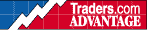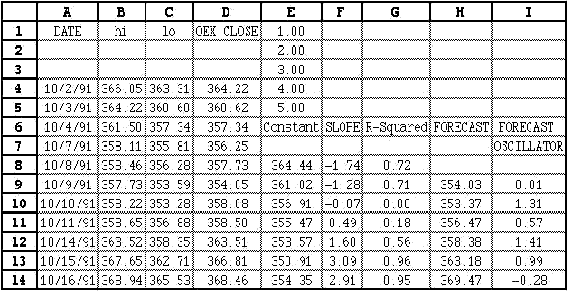HOT TOPICS LIST

INDICATORS LIST

LIST OF TOPICS

# Setting Up an Excel Spreadsheet for the Forecast Oscillator

10/26/00 03:49:17 PM
by Dennis D. Peterson

Security:   N/A
Position:   N/A

 Following is an example of setting up an Excel spreadsheet for creating a forecast oscillator using a five-day linear regression for tomorrow's close. Figure 1, Column A, lists the dates and Column D lists the closing prices (the Y variables) used for the forecasted close. Cells El through E5 are the constants used for the X variables.Figure 1: Sample Excel spreadsheet for creating a forecast. An Excel spreadsheet has built-in formulas to calculate the estimated coefficients m and c of a linear regression and the r-squared value. For example, the formula in cell E8 is: =INDEX(LINEST(D4:D8,\$E\$1:\$E\$5),2)This formula is calculating coefficient c, which is our constant based on the last five days' data. Cell F8 returns the value of the slope m:=INDEX(LINEST(D4:D8,\$E\$1:\$E\$5),1)The formula for cell G8 returns the r-squared:=INDEX(LINEST(D4:D8,\$E\$1:\$E\$5,TRUE,TRUE),3)The key is the last value showed in the formula before the final parenthesis, as this instructs the spreadsheet to either return c, m, or r-squared.Once you have the first set of coefficients, you can forecast tomorrow's close. This is performed in cellH9:=E8 +F8*6Finally, the formula for the forecast oscillator is listed in I9:=(D9-H9)/D9*100--Editor

Dennis D. Peterson

Market index trading on a daily basis.

 Comments or Questions? Article Usefulness 5 (most useful) 4 3 2 1 (least useful)